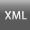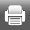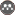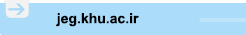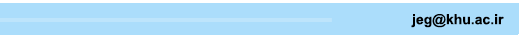[Home ] [Archive]   [ فارسی ]Main About Current Issue Archive Search Submit ContactVolume 14, Issue 1 (5-2020)JEG 2020, 14(1): 53-78 Back to browse issues page
Bearing Capacity of Strip Foundation on Slope Based on Finite Element Limit Analysis Method
1- , Aminpour.geoeng@gmail.com
Abstract:   (541 Views)
Introduction
Studying the effect of slope angle on bearing capacity of foundations on the slope in urban areas is a challenging problem that has been investigated by researchers for years. In general, the analytical approaches for solving this problem can be categorized into limit equilibrium, characteristics and limit analysis methods. In recent years, there have been studies for using the limit analysis within the framework of finite element method for geomaterials. In these studies, the soil mass is not considered as rigid and there is no need to predefine a failure surface for the slope. In the performed research, using the upper bound finite element limit analysis, bearing capacity of strip foundation on slope have been studied. This analytical method enables the use of the advantages of both methods of limit analysis and finite element analysis. In this method, the slip between the two elements is considered. In order to find the critical state of the failure, the rate of power internally dissipated is linearly optimized, by using the interior points method. The advantages of this method are the high convergence rate in comparison with other analytical optimization methods. The effect of different upstream and downstream slopes and foundation depths and also the influence of various mesh discretizations have been evaluated. Finally, the results are compared with those obtained from previous methods available in the literature.
Methods
The finite element limit analysis method is based on nodal velocities. Considering the principals of the finite element method and having the nodal velocities, the velocity at each node of the element can be obtained from corresponding shape functions. The rate of power internally dissipated in each element is defined by multiplying the strain rate on stress in each element. In this method, the slip between the two elements and the rate of internal power dissipated at each discontinuity of two adjacent elements is considered. For this purpose, in each node, four new unknowns’ velocities are defined. To remove the stress from the equations, and provide a linear relationship for linear optimization, a linear approximation to the yield function has been used. For this purpose, the Mohr-Coulomb yield criterion is estimated with a polygon in the stress space. Also, using the reduced strength parameter, the effect of the dilation angle is considered. According to the principles of upper bound limit analysis, the value of plastic strain rate is calculated from the flow rule. The velocity field in elements and discontinuities must satisfy the set of constraints imposed by an associated flow rule. In order to have an acceptable kinematics field, the velocity vectors have to satisfy the boundary conditions. These conditions include zero kinematics velocities along the vertical and horizontal boundaries of the geometry as well as negative vertical unit velocities and zero horizontal velocities at points underneath the rigid foundation.
Results and discussion
In order to calculate the bearing capacity of foundation, a set of different uniform and non-uniform mesh has been examined. The results obtained from different uniform mesh sizes indicate a certain divergence in the course of analysis. However, the results between the fine and very fine non-uniform mesh are closely related to each other and are converged. The obtained results show that, by increasing the internal friction angle, the bearing capacity has been increased. At high angles of modified friction, the effect of increasing the internal friction angle on the increase in bearing capacity is more in slopes with lower angles. By increasing the downstream foundation depth, the bearing capacity has been increased. This increase is more important in the case of slopes with lower angles. However, the upstream depth variations didnchr('39')t present a significant effete on bearing capacity. In order to investigate the effect of upstream angle on the bearing capacity, the upstream mesh is also refined similar to the downstream. The obtained results indicate that variations of the upstream angle have a minor effect on the bearing capacity. This is of course true if the upstream slope is fully stable. The results of the proposed method in this study are an upper bound for the results reported by the limit equilibrium and displacement finite element methods. As seen in Figure 1, the suggested method predicts lower bearing capacities compared to rigid block limit analysis method and is indeed a lower bound for the classical limit analysis method. The finite element limit analysis with linear optimization has resulted in more bearing capacity than cone optimization. The bearing capacities, obtained from characteristic lines method depending to the slope angles, in some cases is more and in some cases less than those explored by the proposed method.
In this paper, the bearing capacity of foundation located on slope was evaluated by finite element limit analysis method. In this regard, the effects of different downstream and upstream angles of slope and foundation depths and also, the effect of various mesh discretizations on the bearing capacity were studied. It is shown that an increase in the downstream angle causes a decrease in the bearing capacity and an increase in the downstream foundation depth leads to an increase in the bearing capacity.  However, the upstream angle and upstream foundation depth were not much effective on the bearing capacity.

Keywords:  bearing capacity, strip footing on the slope, finite element method, limit analysis method, upstream and downstream slopes variations
Type of Study: Research Paper | Subject: Geotecnic
Received: 2018/10/12 | Accepted: 2019/01/5 | Published: 2020/06/9